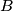### IMO Shortlist 1999 problem G3

Kvaliteta:
Avg: 0,0
Težina:
Avg: 7,0
A set$S$ of points from the space will be called completely symmetric if it has at least three elements and fulfills the condition that for every two distinct points$A$ and$B$ from$S$, the perpendicular bisector plane of the segment$AB$ is a plane of symmetry for$S$. Prove that if a completely symmetric set is finite, then it consists of the vertices of either a regular polygon, or a regular tetrahedron or a regular octahedron.
Izvor: Međunarodna matematička olimpijada, shortlist 1999